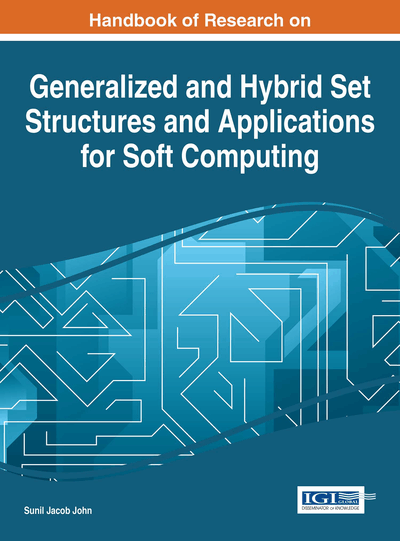# Generalized Rough Sets

Mona Hosny (Ain Shams University, Egypt), Ali Kandil (Helwan University, Egypt), Osama A. El-Tantawy (Zagazig University, Egypt) and Sobhy A. El-Sheikh (Ain Shams University, Egypt)
DOI: 10.4018/978-1-4666-9798-0.ch011

## Abstract

This chapter concerns construction of a new rough set structure for an ideal ordered topological spaces and ordered topological filters. The approximation space approached depend on general binary relation, partially order relation, ideal and filter concepts. Properties of lower and upper approximation are extended to an ideal order topological approximation spaces. The main aim of the rough set theory is reducing the bouwndary region by increasing the lower approximation and decreasing the upper approximation. So, in this chapter different methods are proposed to reduce the boundary region. Comparisons between the current approximations and the previous approximations (El-Shafei et al.,2013) are introduced. It's therefore shown that the current approximations are more generally and reduce the boundary region by increasing the lower approximation and decreasing the upper approximation. The lower and upper approximations satisfy some properties in analogue of Pawlak's spaces (Pawlak, 1982). Moreover, we give several examples for comparison between the current approach and (El-Shafei et al., 2013).
Chapter Preview
Top

## 1 Introduction

In early of 1982, Pawlak (Pawlak, 1982) had proposed rough set theory. It has achieved a large amount of applications in various real-life fields, like economics, medical diagnosis, biochemistry, environmental science, biology, chemistry, psychology, conflict analysis, medicine, pharmacology, banking, market research, engineering, speech recognition, material science, information analysis, data analysis, data mining, linguistics, networking and other fields can be found in [Koaze et al., 2013, Lee et al. 2006, Pal et al. 2014].

The standard rough set theory was based on an equivalence relation on a finite universe X. Various generalized rough set models have been established and their properties or structures have been investigated intensively [Abo–Tabl, 2010, Kozae et al., 2010, Yao,1998,1999]. An interesting and natural research topic in rough set theory is to study rough set theory via topology [Abo Khadra et a1., 2007, Kondo et al., 2006, Kozae et al., 2007, Kozae et al., 2013].

Rough set is dealing with vagueness (ambiguous) of the set by using the concept of the lower and upper approximations (Pawlak, 1982). The set with the same lower and upper approximations, called crisp (exact) set, otherwise known as rough (inexact) set. The boundary region is defined as the difference between the upper and lower approximations, and then the accuracy of the set or ambiguous depending on the boundary region is empty or not respectively. A nonempty boundary region of a set means that our knowledge about the set is not sufficient to define the set precisely. The main aim of rough set theory is reducing the boundary region by increasing the lower approximation and decreasing the upper approximation.

Order theory is a fascinating and natural subject that provides a unified perspective on many areas in mathematics. The concept of ordering plays an important role in different fields of applications (Rassafi et al., 2006) and it is still an active area of research. One of the basic concepts in order theory is that of a partial order, it formalizes the notion of a hierarchy and it appears everywhere in mathematics.

Ordering is one of the most basic concepts in human thinking. Two objects are ordered by simple comparison, the basis of the comparison relies on rule that determines which item is bigger. This rule for comparing two objects is called a binary ordering relation. This activity can be extended to a set of objects by considering all pairwise comparisons. These pairwise comparisons will result in a partially ordered set. We say that the set is partially ordered because it is possible for two objects to be incomparable according to the binary ordering relation we have chosen.

The importance of ordered structures has been increasingly recognized recently because of an explosion of interest in computer science and all areas of discrete mathematics.

The original rough set theory does not consider attributes with preference-ordered domains, that is, criteria. In fact, in many real-world situations, we are often faced with the problems in which the ordering of properties of the considered attributes plays a crucial role. Recently, El-Shafei et al. (EL-Shafei et al., 2013) had studied rough set theory via ordered topology. The study of order relations in topological spaces was initiated by Nachbin (Nachbin, 1965) in 1965. A topological ordered space (X,τ,ρ) is a set X endowed with both a topology τ and a partially order relation ρ on X. A topological ordered space (X,τ,ρ) is a generalization of the study of general topological space. Every topological ordered space (X,τ,ρ) can be regarded as a topological space (X,τ) if ρ is the equality relation “∆”.

## Complete Chapter List

Search this Book:
Reset